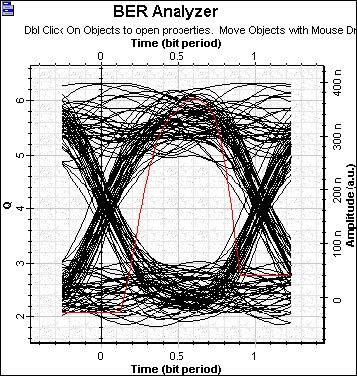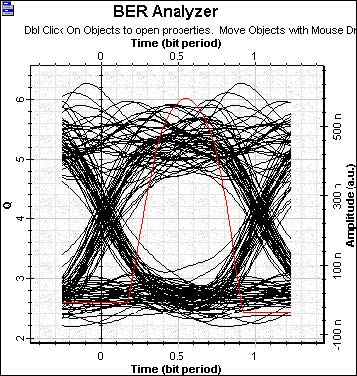This example shows the minimum optical power that a receiver needs to operate reliably with a BER below a specific value (see Figure 1). In this example, you calculate this input power by targeting a BER of 10-9, a Q factor equal to 6 for a PIN photodetector, and an APD.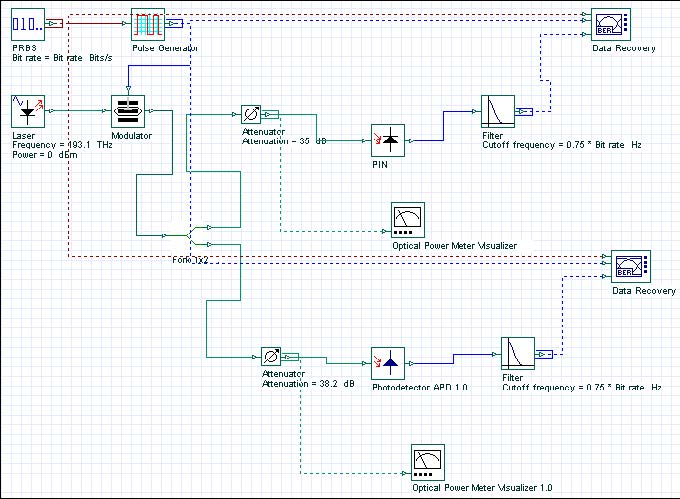This example shows that the receiver sensitivity by using the PIN -32.8 dBm (see Figure 2) and the APD with a gain equal to 3, the sensitivity increases to -41.4 dBm (see Figure 3).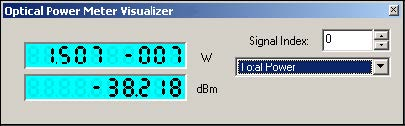Figure 2: Power PIN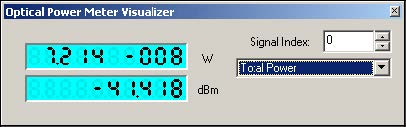Figure 3: Power APD